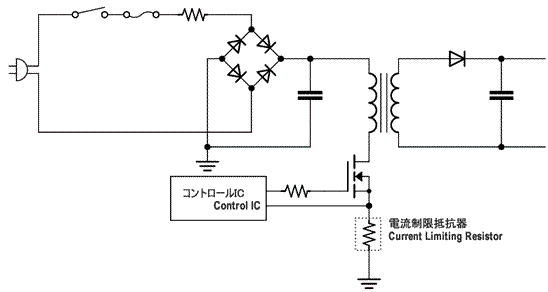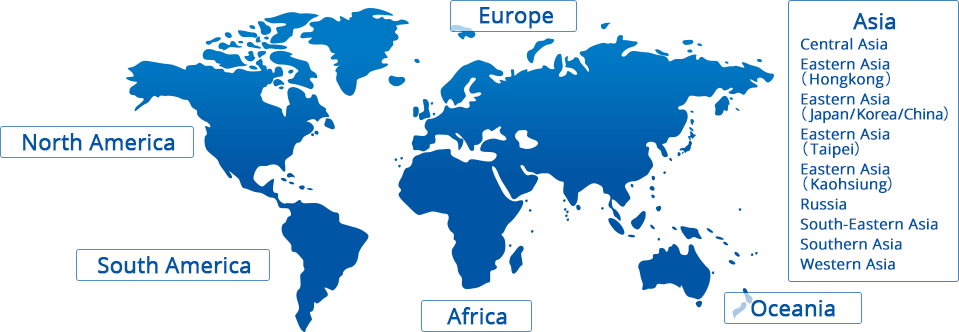# Products# Current Sense Resistors

## Principle of Current Sense

When current is applied to a resistor, voltage drops at both end of the resistor according to Ohm's law. You will get the current value applied to the resistor when you divide the voltage by resistance value. When you adjust the resistance large to make voltage drop large, heating of the resistor would be an issue. To avoid this, current sense should be achieved by using small resistance as possible. When the voltage drop is small, the voltage should be amplified by amplifying circuit.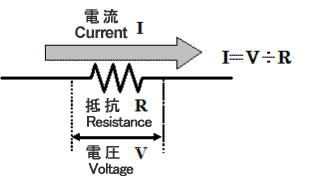## Purposes of Current Sense Resistors

The purposes of current sense in a circuit are divided into following three ways;

### Overcurrent sensing

Circuit operation needs to be stopped for its safety when overcurrent flows by circuit malfunction or overload status. If the current exceeding the specified value is flowed, the status would be considered to be abnormal and the output is interrupted.

### Current control

Detects the peak of saw-toothed waveform current flowed in reactor of DC/DC converters and uses for control.
To rotate three-phase motor, the phase and time of current should be controlled precisely. So this types of product detects the current flowed to each phase and gives feedback to the control circuit. Used mainly for EPS(electric power steering) or air conditioners.

### Current Management(Battery Management)

Portal devices operated by secondary battery detect current or voltage applied to the batttery and grasp the remaining level and controls the circuit operations properly to endure to be used for long time.

Purpose How it works Application examples
Overcurrent Detection Stops circuit operation to keep safe
when overcurrent flows by circuit malfunction.
• Overcurrent protection of power supply circuit
• Over discharge/overcharge protection of secondary battery
• Overcurrent protection of motor
Current Control Detects current and feedback to control circuit in order to control current flowed to circuit, time and phase.
• DC-DC converter
• Inverter power supply
• Current control of AC motor
Current Management By detecting charge/discharge current of mobile devices in real time, to understand the remaining level of secondary battery and to achieve the proper operation of power supply circuit.
• Current management of notebook PC and mobile devices driven by secondary battery drive
• Current management of secondary battery of hybrid vehicles.

## Categories of current sense resistors

Generally, the resistance of current sense resistor should decrease the voltage from a few tens mV to a few hundreds mV when current flows. Current sense low ohm resistors apply low resistance at a few ohms or under. The resistance depends on the current value that you want to detect.
To detect large current of a few tens A, the extreme low resistance of a few milliohm is required. Metal plate type or metal foil type low ohm resistors with small resistance are mainly used.
Thick film type resistors are used to detect low current by using comparatively large resistance like a few hundreds miliohm to a few ohm. See our product lineup of current sense low ohm resistors. Thick film resistors are used to detect small current because relatively large resistance from a few hundreds of milliΩ to a few ohm is used for detecting.

To detect overcurrent, metal film type resistors MF or MOSX are also used.

## Precautions to sense large current

Low ohm resistors uses extremely low resistance of a few miliohm to detect large current. To detect current precisely, pay attention to;

• Layout of voltage detecting patterns
• Inductance of low ohm resistors(if the temporal dI/dt change of current is large)

### The layout of voltage detecting patterns

To detect voltage drop when current is flowed to a resistor, the layout of voltage detecting patterns should be designed from both ends of the resistor.
Voltage detecting pattern is recommended to be designed from the center of electrode pad of resistor as image 2 below. This is to avoid the influence from the voltage drop by the resistance of Cu foil pattern, because Cu foil pattern of circuit board has small resistance.
If the voltage detecting pattern is designed from the side of the electrode pads as the image 1 below, voltage drop is detected with the influence of the resistance of low ohm resistor added by resistance of Cu foil pattern, which unables precise current detection.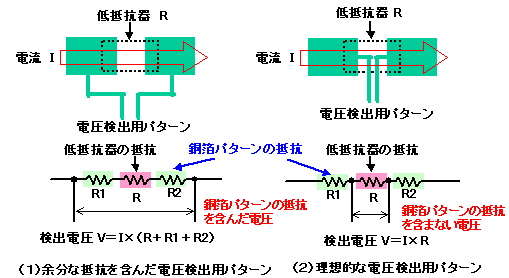### Inductance of low ohm resistors

As explained for frequency characteristics of resistors, resistor is more likey affected by parasitic inductance when the resistance is low, and the impedance increases when the frequency gets higher. DC current gets no influence but for AC current like square waveform including high frequency components, precise detection is impossible.
When AC current flows as the image below, the voltage decrease would be the total sum of the one by resistance and the one by inductance. Thus, the low ohm resistor with minimum inductance should be used for large current sensing.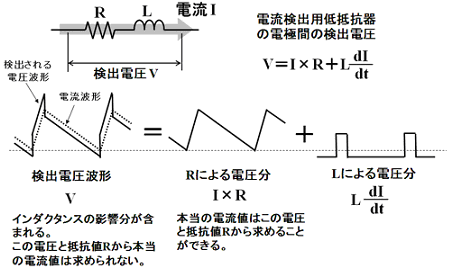## Four-terminal current sense resistors

Current sense low ohm resistors include four-terminal type, with voltage detecting terminals to detect voltage and current terminals to flow current. Below is the example of four-terminal type current sense low ohm resistors. The advantage of four-terminal is to enable to detect current more precisely, with less error of the detected value by the voltage detecting pattern layout.
The disadvantage is the total resistance becomes larger than two-terminal type because its construction makes it easier to produce resistance between voltage detecting terminal and current terminal as well as the resistance to detect current. Compared to two-terminal type, the cunsumed power is large when the same current is flowed and the heat generation of the resistor becomes large. If the resistance for detecting large current is a few milliohm, the resistance between voltage detecting terminal and current terminal affects largely, leading to much more wasted power consumption. Also, by its construction, parasitic inductance gets larger, which is not suitable for sensing current where current change dI/dt is large.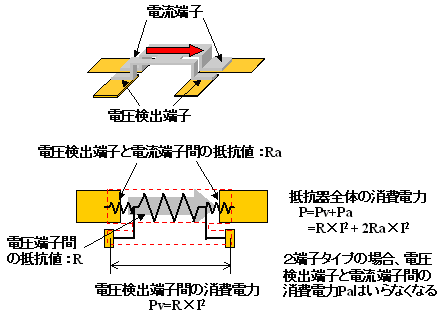## Overcurrent sensing

Current sense low ohm resistors are sometimes used for transistor source(or emittor) to detect overcurrent of switching transistor. This resistor is required to be free of smoking or firing even if the transistor breaks down and large current is applied. The inductance component is required to be low. Metal type low ohm resistors BPR, SL, TSL, LR72 and LR are recommended for current sense low ohm resistors.